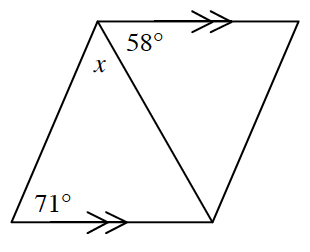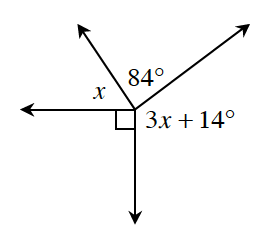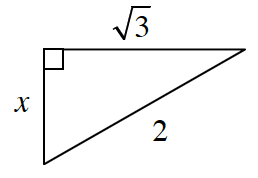### Home > CCG > Chapter 3 > Lesson 3.2.3 > Problem3-78

3-78.

Use the relationships in each diagram below to solve for $x$. Justify your solution by stating which geometry relationships you used.

1.Parallel lines cut by a transversal creates equal angles.Which angles in the figure are equal?

1.Think of the angle measure of a circle. How can you use that measure to solve for $x$?

$360º=x+3x+14º+84º+90º$

1.Use the Pythagorean Theorem. Remember to justify all your work with the relationships you used!